# Thermodynamic Analysis of Window Air Conditioner Using Sustainable Refrigerant R290/RE170 and R1270/RE170 Blends as Substitutes to Refrigerant R22

Thermodynamic Analysis of Window Air Conditioner Using Sustainable Refrigerant R290/RE170 and R1270/RE170 Blends as Substitutes to Refrigerant R22
Department of Mechanical Engineering, National Institute of Technology Karnataka, Surathkal-575025, Mangalore, India

Corresponding Author Email:
sharmasvali.nitk@gmail.com
Page:
80-94
|
DOI:
https://doi.org/10.18280/ijht.370110
19 May 2018
|
Accepted:
9 March 2019
|
Published:
31 March 2019
| Citation

OPEN ACCESS

Abstract:

The present work emphasis on theoretical computation of thermodynamic performance of window air conditioner using various sustainable R290/RE170 and R1270/RE170 refrigerant mixtures as substitutes to R22. In this work, apart from R407C, twelve new binary mixtures comprising of R290, R1270 and RE170 at various compositions were developed. And also in this investigation, a MATLAB code was developed to compute the thermodynamic performance characteristics of various considered R22 alternatives at Tk=54.4 ℃ and Te=7.2 ℃. The various performance characteristics computed are mass flow rate, refrigeration effect, compressor work, coefficient of performance (COP), pressure ratio, compressor discharge temperature, power consumed per ton of refrigeration, condenser heat rejection and volumetric cooling capacity. Results showed that the COP of refrigerant mixture RM7 (R1270/RE170 95/5 by mass %) was the highest among twelve refrigerants studied and it was 0.23 % higher than R22. Pressure ratio of RM7 (3.174) was 7.49 % lower than that of R22 (3.431). Compressor discharge temperature of all the twelve investigated refrigerants was lower in the range of 9.35 ℃ to 17.15℃ when compared with R22. Power consumed per ton of refrigeration of RM7 was 0.27 % lower than that of R22. Volumetric cooling capacity of RM7 (3833 kJ/m3) was very close to that of R22 volumetric capacity (3863 kJ/m3). Heat transfer through condenser of RM7 (6.372 kW) was similar to that of R22 (6.377 kW).  Overall, thermodynamic performance of RM7 matches well with the performance of base line refrigerant R22 and hence, refrigerant RM7 can be considered as sustainable alternative to R22 used in air conditioners.

Keywords:

COP, discharge temperature, R22 alternatives, sustainable refrigerants, volumetric cooling capacity

1. Introduction

The ongoing phase out schedule of ozone depleting substance like R22 needs the development of ecofriendly refrigerants, since Hydrochlorofluorocarbon (HCFC) refrigerant R22 causing significant ozone layer depletion and high global warming in the atmospheric environment due to the presence of chlorine atoms in R22 . Therefore, Montreal protocol have decided to phaseout R22 by the year 2030 in all the nations [2, 3]. In this context, many nations are keenly focusing on the development of their own R22 alternatives. Earlier, various experimental and theoretical performance studies were conducted in order to find viable alternatives to R22.

Experimental investigation on air-to-water heat pumps was carried out with different refrigerants including R22, R134a and R404A in order to find the optimal refrigerant charge . Results showed that the overcharging and undercharging of refrigerant tends to decrease the performance of heat pump. Theoretical performance investigation was done with various refrigerants like R134a, R430A, R440A and R450A used in domestic refrigerator . Results revealed that the coefficient of performance (COP) of R440A was the highest among R134a, R430A and R450A. Experimental performance assessment of air conditioner was done with R410A under various indoor working conditions . Results showed that the performance of air conditioner with R410A was better under wide range of operating conditions.

An extensive study reported that refrigerant R32 was recommended as a viable candidate to replace R410A used in air conditioners . Segment-to-Segment modeling of microchannel heat exchangers (condenser and evaporator) was developed, in order to predict the performance of air conditioning system using various alternative refrigerants . The benefit of microchannel heat exchangers was it reduces the charge of refrigerant required to the system. From this modeling of microchannel heat exchangers, it was observed that R32 shows the better performance compared to R410A whereas R22 and R290 had similar performance, however R290 required a larger displacement volume of compressor.

Theoretical performance investigation was conducted on simple vapour compression refrigeration (VCR) system working with R432A and R433A as alternatives to R22 . Results exhibited that the performance of R432A and R433A was closer to refrigerant R22. Experimental studies revealed that R407C was considered as an appropriate retrofit refrigerant to R22 . Theoretical analysis of exergy revealed that R507A was an appropriate refrigerant to substitute R502 rather than R404A . Theoretical performance investigation was done on simple VCR system working with R134a, RE170 and R510A, under various operating conditions . Results showed that the COP of RE170 and R510A was better than R134a.

Experimental performance tests were conducted in a vapour compression system of 5TR capacity using both variable speed compressor and electronic expansion valve, operating at different evaporator temperatures. In this investigation, refrigerants tested were R22, R32, R438A, R404A, R410A, R290 and R1270 . Test results shown that COP of R290 and R1270 was better than R22 and other investigated refrigerants. Experimental and theoretical investigations were done in air conditioner of 3.5kW capacity working with R22 and R161 under various operating conditions . Results revealed that the performance of R161 was better than refrigerant R22. Experimental investigation on ice cream refrigerator was done with R404A and its alternative refrigerant mixture R290/RE170 . Test results shown that the time taken to form ice cream for the mixture R290/RE170 (65/35 by mass %) was less compared to R404A and also its COP was better than R404A. Experimental studies were conducted in a heat pump bench tester with R22 and R432A (R1270/RE170 80/20 by mass %) under both the cooling mode (air conditioning) and heating mode (heat pumping) conditions . Test results exhibited that the COP of R432A was higher in the range of 8.5 to 8.7%, compared to R22 in both the conditions.

A comprehensive analysis of an automotive condenser under maldistribution of intake air was carried out by both experimental and numerical simulations . Simulation studies were done using Coil Designer software, in order to simulate the heat transfer and fluid dynamics of cross flow heat exchanger (condenser), whereas experiments were carried out in a condenser of a stationary test facility for an automotive air conditioning system over a wide range of blockage conditions. In this study, the experimental results exhibited good agreement with the simulation results, which assures the applicability of simulation results for situations where experimental results were not available. From this investigation it was also observed that, in case of the blocked condition, the heat exchange zone was extended to a longer length depending upon the category and magnitude of the blockage. Experimental performance study and exergy analysis was carried out with R1234yf used in automotive air conditioning system . In this study, the performance of R1234yf systems with and without internal heat exchanger (IHX) was compared with an R134a system. Results showed that COP of R1234yf system without IHX was 3.6 to 4.5 % lower compared to the R134a system, whereas COP of R1234yf system with IHX was 0.9 % higher compared to the R134a system at the compressor speed of 2500 rpm. The exergy destruction ratio (EDR) of R1234yf system with IHX was 1.2 % lower compared to the R134a system at the compressor speed of 2500 rpm.

Experimental performance tests were conducted with low global warming potential (GWP) refrigerant blend R1234yf/R134a (89/11 by mass %) as substitute to R134a under both the cooling and heating modes respectively . Results revealed that, capacities of R1234yf/R134a (89/11 by mass %) and R134a were similar in both cooling and heating modes.  The COP of R1234yf/R134a (89/11 by mass %) was 4 to 16% lower than R134a in heating mode, whereas it was 4 to 9% lower than R134a in cooling mode. Experimental test results revealed that the refrigerant mixture R290/R600/R600a (50/40/10 by mass %) would be considered as a suitable replacement to R134a used in automotive air conditioners, since it has higher COP than R134a in all the tested conditions and also it has similar working temperature and pressure as that of R134a . A literature review revealed that hydrocarbons and their mixtures would be considered as suitable alternatives to halogenated refrigerants . A literature review reported that R717 and R744 were considered as feasible refrigerants for industrial applications whereas R290, R600a, R1150 and R1270 were considered as viable refrigerants for air conditioning applications .

From the literature, it is noticed that the refrigerant Dimethylether (RE170) is considered as a viable ecofriendly refrigerant and it can be used as a blending component with different refrigerants in order to reduce the global warming potential (GWP) as well as to enhance the COP of refrigerant blend. Therefore, the present study focuses on theoretical thermodynamic performance investigation of standard vapour compression refrigeration system working with various sustainable refrigerant blends like R290/RE170 and R1270/RE170 as substitutes to R22 used in air conditioners. And also in this study, thermodynamic performance results found from the MATLAB code have been validated with the results published in the literature.

2. Development of New Alternative Refrigerant Blends and Its Properties

In this work apart from R407C, twelve new binary refrigerant blends comprising of R290, R1270 and RE170 at various compositions were developed. Environmental properties like ozone depletion potential (ODP) and global warming potential (GWP) of various considered refrigerants were taken from the ASHRAE data hand book . The designation of refrigerant blends that were developed in this study is given in Table 1. Similarly basic properties of all the considered R22 alternative refrigerants are given in Table 2.

Table 1. Designation of various developed alternative refrigerants

 Designation of refrigerants Composition (mass %) R22 Pure refrigerant RM1 (R290/RE170) 95/5 RM2 (R290/RE170) 90/10 RM3 (R290/RE170) 85/15 RM4 (R290/RE170) 80/20 RM5 (R290/RE170) 75/25 RM6 (R290/RE170) 70/30 RM7 (R1270/RE170) 95/5 RM8 (R1270/RE170) 90/10 RM9 (R1270/RE170) 85/15 RM10 (R1270/RE170) 80/20 RM11 (R1270/RE170) 75/25 RM12 (R1270/RE170) 70/30 R407C (R32/R125/R134a) 23/25/52

From Table 2, it is noticed that all the twelve developed refrigerant mixtures (RM1 to RM12) possess zero ODP and very low GWP compared to R22. Therefore refrigerants (RM1 to RM12) can be considered as ecofriendly refrigerants. From Table 2, it is also observed that the refrigerant mixtures (RM1 to RM4) are considered as near azeotropic due to their lower temperature glide (less than 0.5 ℃), whereas refrigerants (RM5 to RM12 and R407C) are categorized into non azeotropic mixtures, since these refrigerants possess higher temperature glide.

Table 2. Basic properties of developed alternative refrigerants

 Refrigerants MW (kg/kmol) BP (0C) Tbub (0C) Tdew (0C) T*glide (0C) Tc (K) Pc (MPa) ODP GWP (100 years) R22 86.5 -40.81 0 0 0 369.3 4.99 0.055 1760 RM1 44.19 - -41.99 -41.97 0.02 370.13 4.287 0 <3 RM2 44.285 - -41.76 -41.67 0.09 370.48 4.325 0 <3 RM3 44.381 - -41.42 -41.20 0.22 370.97 4.365 0 <3 RM4 44.477 - -40.93 -40.47 0.46 371.59 4.407 0 <3 RM5 44.573 - -41.11 -40.30 0.81 372.36 4.453 0 <3 RM6 44.669 - -39.51 -38.23 1.28 373.29 4.502 0 <3 RM7 42.263 - -46.36 -45.68 0.68 365.69 4.604 0 <3 RM8 42.447 - -45.07 -43.69 1.38 367.22 4.654 0 <3 RM9 42.633 - -43.76 -41.69 2.07 368.79 4.705 0 <3 RM10 42.821 - -42.45 -39.71 2.74 370.41 4.756 0 <3 RM11 43.011 - -44.53 -41.15 3.38 372.06 4.807 0 <3 RM12 43.202 - -39.86 -35.88 3.98 373.76 4.858 0 <3 R407C 86.204 - -43.62 -36.62 7.0 359.18 4.631 0 1774

T*glide = (Tdew-Tbub) at 0.101325MPa

2.1 Development of properties of alternative refrigerants

Generally, thermodynamic properties of refrigerants are essential to compute performance characteristics of R22 and its various considered alternatives. Therefore, in this work, thermodynamic properties of all the considered R22 alternative refrigerants were developed with the help of a Martin-Hou equation of state (MHEOS). The significance of MHEOS is that, it gives better accuracy in results while computing thermodynamic properties of refrigerants [24-26]. Literatures on applicability of MHEOS were discussed below.

Experimental studies were conducted using a constant volume apparatus, in order to measure the vapor phase PvT Properties of refrigerant R1225ye(Z) over temperature ranges from (263 to 368) K and pressure ranges from (135 to 777) kPa . In this study, the experimental vapour phase PvT Properties of R1225ye(Z) were compared with a Martin-Hou equation of state (MHEOS). Results showed that the deviations between computed values of PvT using MHEOS and experimental values were (-0.54 to 0.52) % with an average absolute deviation (AAD) of 0.170. Experimental tests were performed using a constant volume apparatus, in order to measure the vapor phase PvT Properties of refrigerant R1243zf over temperature ranges from (268 to 368) K and pressure ranges from (220 to 910) kPa . In this work, the experimental vapour phase PvT Properties of R1243zf were compared with a Martin-Hou equation of state (MHEOS). Results revealed that the deviations between computed values of PvT using MHEOS and experimental values were (-0.65 to 1.27) % with an average absolute deviation (AAD) of 0.305.

Experimental investigations were carried out by using a constant volume apparatus, in order to measure the vapor phase PvT Properties of refrigerant R1234ze(E) over temperature ranges from (243 to 373) K and pressure ranges from (57 to 1024) kPa . In this study, the experimental vapour phase PvT Properties of R1234ze(E) were compared with a Martin-Hou equation of state (MHEOS). Results shown that the deviations between computed values of PvT using MHEOS and experimental values were (-0.35 to 0.38) % with an average absolute deviation (AAD) of 0.198. Experimental tests were done by using a constant volume apparatus, in order to measure the vapor phase PvT Properties of refrigerant HFO1234yf over temperature ranges from (243 to 373) K and pressure ranges from (84 to 3716) kPa . In this study, the experimental vapour phase PvT Properties of HFO1234yf were compared with a Martin-Hou equation of state (MHEOS). Results exhibited that the deviations between computed values of PvT using MHEOS and experimental values were (-1.18 to 1.27) % with an average absolute deviation (AAD) of 0.44.

Dong et al., proposed an empirical correlation, to conveniently compute parameter B4 of the Martin-Hou equation of state (MHEOS) at various temperatures by relating B4(T) with B4(0.7) (B4 at reduced temperature=0.7) after analyzing B4(T) of more than 200 compounds . In this study, the accuracy of MHEOS to compute volume of various refrigerants by using proposed correlation was verified by comparing the calculated data with literature data. An empirical correlation used to compute B4 was given below.

$ln(R_a )=-0.40379×10^{-2}×(V_c-b)×(T_r-0.7)+2.4189×(T_r-0.7)^2$ (1)
$R_a=\frac{B_4(T)}{B_4(0.7)}$ (2)
$T_r=\frac{T}{T_c}$ (3)

Results showed that the proposed equation improves the accuracy in results of properties obtained from the MHEOS and also significantly decreases the computational time to determine parameter B4, which would enhance the application of the MHEOS in engineering calculations.

From the available literature, it is evident that the Martin-Hou equation of state (MHEOS) can be conveniently used in chemical engineering, mechanical engineering, and refrigeration technology design. Therefore, MHEOS was used in the present study to compute thermodynamic properties of various considered R22 alternative refrigerants. The methodology followed to establish thermodynamic properties of refrigerants was explained below.

2.2 Methodology to establish thermodynamic properties of pure and mixture refrigerants

Procedure followed to establish thermodynamic properties of pure and mixture refrigerants was taken from literature and it was discussed below [32, 33].

2.2.1 Methodology to develop properties of pure refrigerants

Correlations used to develop thermodynamic properties of refrigerants are given in this section. Pressure-Enthalpy (P-h) chart used while developing properties of pure refrigerants is shown in Figure 1. Step by step procedure followed to develop the properties of refrigerants is given below.

## fig1.gif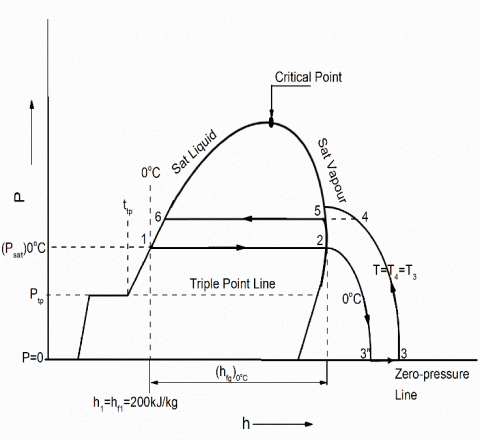Figure 1. P-h chart for computing properties of pure refrigerant

(i) Initially, compute the saturation pressure of given refrigerant. The correlation between saturation pressure (Psat) and saturation temperature (Tsat) was given by Wagner saturation vapour pressure equation and it is given below .
$ln\left(\frac{P_{sat}}{P_c}\right)=\left(\frac{1}{1-x}\right)[AX+Bx^{1.5}+Cx^{2.5}+Dx^5]$ (4)

where x=1-T/Tc; A, B, C and D are constants for a particular refrigerant and these constants for several refrigerants were available in the literature . For example, saturation vapour pressure constants for refrigerant R22 are given in Table 3.

Table 3. Constants of R22 for equation (4)

 A B C D -7.0682 1.52369 -1.8545 -2.8439

(ii) Compute the liquid density of given refrigerant. In the present study, Reid et al., correlation was used to find the liquid density of various refrigerants .

$\rho_r=\frac{\rho}{\rho_c} =1+0.85*(1-T_r )+(1.6916+0.984*ω)*{(1-T_r)}^{1/3}$(5)

where ω is acentric factor; Tr=T/Tc, ω, ρc and Tc are constants for a particular refrigerant and these constants for several refrigerants were available in the literature . For example, density correlation constants for refrigerant R22 are given in Table 4.

Table 4. Constants of R22 for equation (5)

 ω ρc (kg/m3) Tc (K) 0.221 523.8 369.15
(iii) Compute the specific volume of vapour for a given refrigerant. In the present work, specific volume of vapour for all the considered alternative refrigerants was computed by using Martin-Hou equation of state (MHEOS) [24, 31].

$P=\frac{R T}{v-b}+\frac{A_{2}+B_{2} T+C_{2} e^{\frac{-5.475 T}{T_{C}}}}{(v-b)^{2}}+\frac{A_{3}+B_{3} T+C_{3} e^{\frac{-5.475 T}{T_{C}}}}{(v-b)^{3}}+\frac{A_{4}}{(v-b)^{4}}+\frac{B_{5} T}{(v-b)^{5}}$ (6)

where

$b=V_c-\frac{\beta V_c}{15Z_c}$ (7)

$\beta=-31.883 Z_{C}^{2}+20.533Z_C$ (8)

$Z_C=\frac{P_cV_c}{RT_c}$ (9)

where A2, A3, A4, B2, B3, B5, C2, C3 and b are dimensionless coefficients of MHEOS for various refrigerants.  Procedure followed to compute above coefficients was described in the literature [24, 31]. By solving the above equation (6), dimensionless coefficients of MHEOS for R22 are listed in Table 5.

Table 5. Dimensionless coefficients of R22 for equation (6)

 Dimensionless coefficients of R22 Values A2 -139.154038231457 A3 0.295289024195263 A4 -0.000104165697806786 B2 0.128645931301646 B3 -0.000446322328392750 B5 8.14900447033360 x10-11 C2 -2292.28498497122 C3 3.44337587584321 b 0.000407841281333333

(iv) Compute enthalpy of vapourization of given refrigerant. Clausius-Clapeyron equation was used to find the enthalpy of vapourization of various considered alternative refrigerants.
$\frac{dP_{sat}}{dT}=\frac{h_{fg}}{T \times V_{fg}}$ (10)
where $V_fg= v_g-v_f$

(v) Compute enthalpy and entropy properties (both liquid and vapour phase) of given refrigerant by using departure method. Generally, the reference state of enthalpy and entropy was fixed while computing properties. İn case of refrigerants, the reference state chosen is that of saturated liquid at 0 ℃. Enthalpy and entropy values assigned to the reference state of saturated liquid at 0 ℃ are usually h1=hf1=200 kJ/kg and S1=Sf1=1.0 kJ/kg K respectively [32, 33].

The significance of departure function is, to compute the enthalpy and entropy at various points as shown in Figure 1. For example, to compute enthalpy at point 3, enthalpy departure method is applied and corresponding departure term (h3-h2) is given as follows.

$h_3-h_2=(U_3-U_2 )+(P_3 V_3-P_2 V_2 )$(11)
${{U}_{3}}-{{U}_{2}}=\int\limits_{2}^{3}{\left[ T{{\left[ \frac{\partial P}{\partial T} \right]}_{V}}-P \right]}dV$ (12)

By solving above equations (11) and (12), the value of h3 can be found. In order to find the enthalpy h4 at point 4, ideal gas heat capacity correlation and enthalpy difference (h4-h3) can be used and it is given below.

${{h}_{4}}-{{h}_{3}}=\int\limits_{3}^{4}{C_{P0}dT}$(13)

In the present work, ideal gas heat capacity $(C_{P0})$ correlation was taken from the literature and it is given below .

$C_P0=H_0+H_1 T+H_2 T^2+H_3 T^3+H_4 T^4$ (14)

where H0, H1, H2, H3 and H4 are constants for a particluar refrigerant and these constants for several refrigerants were avialable in the literature . For example, constants of ideal gas heat capacity correlation for R22 are given in Table 6.

Table 6. Constants of R22 for equation (14)

 CP0 constants Values H0 3.164 H1 10.422 x10-3 H2 1.179 x10-5 H3 -2.650 x10-8 H4 1.222 x10-11
In order to find enthalpy h5, once again enthalpy departure method was applied in between the state points 4 and 5, and corresponding enthalpy departure term (h5-h4) is given below.

$h_5-h_4=(U_5-U_4 )+(P_5 V_5-P_4 V_4 )$ (15)

${{U}_{5}}-{{U}_{4}}=\int\limits_{4}^{5}{\left[ T{{\left[ \frac{\partial P}{\partial T} \right]}_{V}}-P \right]}dV$(16)

By solving above equations (15) and (16), the value of h5 can be found. On the other hand, saturated liquid enthalpy at state point 6 can be found by using the following relation.

$h_5-h_6=h_fg$ (17)

$h_6=h_5-h_fg$ (18)

where hfg can be found by using Clasius-Clayperon equation at a given temperature.

(vi) Find the liquid entropy of given refrigerant. In order to compute the thermodynamic properties (enthalpy and entropy) of any given refrigerant at any given pressure and temperature, the departure method was used, and the corresponding saturated liquid enthalpy and saturated liquid entropy was computed by using the Clausius-Clapeyron equation. Entropy of liquid for any given refrigerant can be calculated as follows.

$S_fg=S_g-S_f$ (19)

$S_f=S_g-S_fg$ (20)

(vii) Entropy of vapour for any given refrigerant can be computed as follows.

$S_{fg}=\frac{h_{fg}}{T_{sat}}$ (21)

$S_{fg}=\frac{h_{g}}{T_{sat}}$ (22)

By following the above methodology, thermodynamic properties of various pure refrigerants can be found.

2.2.2 Methodology to develop properties of mixture refrigerants

Correlations used to develop thermodynamic properties of refrigerant mixtures were given in this section. Pressure-Enthalpy (P-h) chart used while developing properties of refrigerant mixtures is shown in Figure 2.

## fig2.gifFigure 2. P-h diagram for computing properties of refrigerant mixtures

(i) Generally, thermodynamic properties data of pure refrigerants was taken into account, while computing properties of refrigerant mixtures.

(ii) In order to compute the bubble point temperature and dew point temperature of refrigerant mixtures, use the interpolation method by taking saturation temperature and saturation pressure data of pure refrigerants.

(iii) For example, correlation used to compute the bubble point temperature of refrigerant mixture is given below.

$p=x_1 p_1^{sat}+x_2 p_2^{sat}$ (23)

where x1 and x2 are mole fractions of pure components in the liquid phase.

(iv) Similarly, correlation used to compute the dew point temperature of refrigerant mixture is given below.

$p=\frac{p_{1}^{sat}p_{2}^{sat}}{p_{1}^{sat}-y_1(p_{1}^{sat}-p_{2}^{sat})}$ (24)

where y1 and y2 are mole fractions of pure components in the vapour phase.

(v) Mixing rules and binary interaction parameter used while developing and establishing the thermodynamic properties of refrigerant mixtures were taken from the literature and these rules were used to find critical temperature and critical pressure of the refrigerant mixture .

$T_{cm}= y_i^2 T_i+y_j^2 T_j+2y_i y_j T_{cij}$ (25)

$T_{cij}=(1-k_{ij} ) (T_{ci} T_{cj} )^{1/2}$ (26)

$1-k_{ij}=\frac{8(V_{ci}V_{cj})^{1/2}}{(V_{ci}^{1/3}+V_{cj}^{1/3})^{3}}$ (27)

${{P}_{cm}}=({{Z}_{cm}}R{{T}_{cm}})/({{V}_{cm}})={\left( \left( \sum\limits_{i=1}^{n}{{{y}_{i}}{{Z}_{ci}}} \right)(R)\left( \sum\limits_{i=1}^{n}{{{y}_{i}}{{T}_{ci}}} \right) \right)}/{\left( \sum\limits_{i=1}^{n}{{{y}_{i}}{{V}_{ci}}} \right)}\;$ (28)

The binary interaction parameter kij was given by

$k_{ij}=1-\frac{8(V_{ci}V_{cj})^{1/2}}{(V_{ci}^{1/3}+V_{cj}^{1/3})^{3}}$ (29)

(vi) Compute the specific volume of vapour for a given refrigerant mixture. In the present investigation, specific volume of vapour for all the considered refrigerant mixtures was computed by using Martin-Hou equation of state (MHEOS) [24, 31].

(vii) In order to compute the liquid density of refrigerant mixtures for a given pressure, use the interpolation method by taking liquid density properties data of pure refrigerants.

(viii) Similarly departure method was used to find the enthalpy and entropy of various refrigerant mixtures and the procedure used for the departure method was described in the literature [32, 33].

By following the above methodology, thermodynamic properties of various considered R22 alternative refrigerant mixtures were computed. For example, the computed thermodynamic properties of various refrigerants like R22, R407C and RM7 (R1270/RE170 95/5 by mass %) were compared with the properties obtained from NIST REFPROP 9.1 . Results showed that the computed properties show good agreement with NIST REFPROP 9.1. The deviation between computed properties of R22, R407C and RM7 using MHEOS and REPROP properties was within 2% for the given operating conditions. Therefore, the methodology followed to establish properties of R22, R407C and RM7 can be considered as reliable. Hence, the same methodology was followed to develop thermodynamic properties of various new refrigerants considered for the study, since properties of various new refrigerants were not available in the literature.

3. Flammability Study of Alternative Refrigerants

Investigation on flammability of refrigerants is important for researchers while developing the alternative refrigerants. ASHRAE safety standard 34 exhibits that, flammability of refrigerants were classified into various safety groups like nonflammable (ASHRAE A1), weakly flammable (ASHRAE A2) and flammable (ASHRAE A3) groups respectively . From this safety standard, it was found that, refrigerants R22, R134a, R125 and R407C were classified into nonflammable category (A1) whereas R32 was classified into weakly flammable (A2). Similarly refrigerants R290, R1270 and RE170 were classified into flammable group (A3).

Table 7. RF number and flammability group of various investigated refrigerants

 Refrigerants RF Number (kJ/g) ASHRAE Flammability Group RM1 52.01 A3* RM2 51.83 A3* RM3 51.68 A3* RM4 51.56 A3* RM5 51.46 A3* RM6 51.40 A3* RM7 61.02 A3* RM8 60.50 A3* RM9 60.01 A3* RM10 59.53 A3* RM11 59.08 A3* RM12 58.66 A3*

* Estimated values of RF number

Table 8. ASHRAE safety group of various investigated R22 alternative refrigerants

 Refrigerants ASHRAE Safety Group ASHRAE Flammability R22 A1 A1 Nonflammable R134a A1 A1 Nonflammable R125 A1 A1 Nonflammable R32 A2 A2 Weakly flammable R407C A1 A1 Nonflammable R290 A3 A3 Flammable R1270 A3 A3 Flammable RE170 A3 A3 Flammable RM1 A3* A3* Flammable RM2 A3* A3* Flammable RM3 A3* A3* Flammable RM4 A3* A3* Flammable RM5 A3* A3* Flammable RM6 A3* A3* Flammable RM7 A3* A3* Flammable RM8 A3* A3* Flammable RM9 A3* A3* Flammable RM10 A3* A3* Flammable RM11 A3* A3* Flammable RM12 A3* A3* Flammable

*Estimated

However flammability category of various new refrigerant mixtures (RM1 to RM12) considered in this study were not available in the ASHRAE safety standard 34 and hence, refrigerant flammability number (RF number) was used in this investigation for assessing the flammability of new refrigerant blends. RF number shows good agreement with that of ASHRAE safety standard 34 which was used for classifying the refrigerants into various flammability categories . It is reliable to express the hazards of combustion with respect to flammability limits of each refrigerant by using RF number. Based on the values of RF number, refrigerants were categorized into various safety groups . If RF number of refrigerants is less than 30 kJ/g, then they are classified into weakly flammable group (ASHRAE A2) and if it is in between 30 to 150 kJ/g, then they are classified into flammable group (ASHRAE A3). An empirical correlation used for computing the RF number of different refrigerants studied is given below.

$RF=\left\{ {{\left( \frac{U}{L} \right)}^{0.5}}-1 \right\}\times \frac{HOC}{MW}$(30)

By using above correlation, values of RF number of various new refrigerant mixtures (RM1 to RM12) were computed and they are given in Table 7. Similarly, the summary of flammability groups of all the R22 alternative refrigerants studied in this investigation are given in Table 8.

From Table 7, it was found that the flammability category of all the twelve investigated refrigerants (RM1 to RM12) are classified into ASHRAE A3 flammability group, since RF number of these refrigerants was in between 30 to 150 kJ/g.

4. Vapour Compression Refrigeration (VCR) System

Basically, window air conditioners work on the principle of vapour compression refrigeration (VCR) system. The basic representation of VCR system is shown in Figure 3. Normally, VCR cycle consists of four basic processes like isentropic compression, constant pressure condensation, isenthalpic expansion and constant pressure evaporation. In majority of literature, thermodynamic performance analysis of air conditioners was done based on either simple saturation vapour compression refrigeration cycle or standard vapour compression cycle [39-42].

Table 9. Description of simple saturation vapour compression refrigeration cycle

 State points of the cycle Description 1-2 Isentropic compression 2-3 Constant pressure condensation 3-4 Isenthalpic expansion 4-1 Constant pressure evaporation

Table 10. Description of standard vapour compression refrigeration cycle

 State points of the cycle Description 1”-2” Isentropic compression 2”-3” Constant pressure condensation 3”-4” Isenthalpic expansion 4”-1” Constant pressure evaporation 1-1” Degree of superheating 3-3” Degree of subcooling

Pressure-Enthalpy (P-h) diagrams of simple and standard vapour compression cycle are shown in Figure 4a and 4b respectively. Either in simple saturation cycle or standard cycle, pressure losses and heat losses to the surroundings from condensers and evaporators were neglected. Similarly suction line pressure drop, discharge line pressure drop and heat gain or heat losses occur at various devices of the system were neglected for the ease of theoretical computations. Description of various state points of simple saturation cycle is given in Table 9. Similarly, description of various thermodynamic stages of standard vapour compression refrigeration cycle is given in Table 10.

## fig3.gif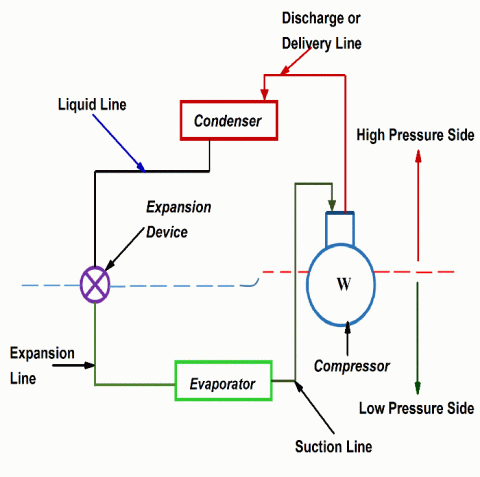Figure 3. Schematic diagram of vapour compression refrigeration system

## fig4a.gif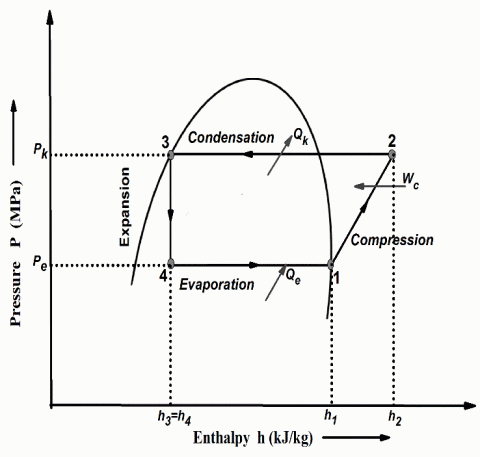Figure 4a. P-h diagram of simple saturation vapour compression refrigeration cycle without superheating and subcooling

## fig4b.gif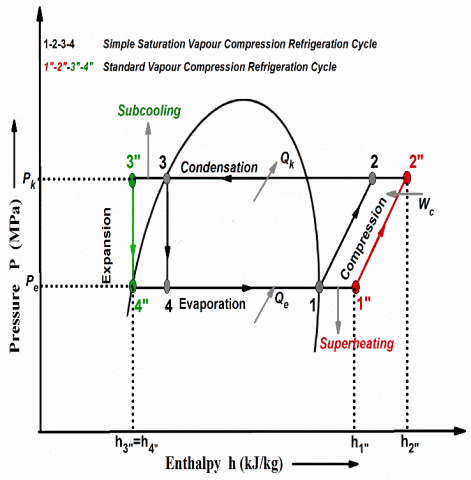Figure 4b. P-h diagram of standard vapour compression refrigeration cycle with superheating and subcooling

In case of simple saturation cycle, effect of superheating and subcooling on performance of the system was not considered, whereas in standard vapour compression cycle, effect of superheating and subcooling on thermodynamic performance of the system was considered. Therefore, the present study focuses on theoretical performance evaluation of air conditioner using various alternative refrigerants based on standard vapour compression refrigeration cycle. Assumptions made, while doing thermodynamic analysis of standard vapour compression system were taken from literature and they are given below [39-42].

(i) Pressure drops in the condenser and evaporator are negligible.

(ii) Heat losses to the environment from the various devices like evaporator and condenser are negligible

(iii) Flow across the expansion valve is isenthalpic.

(iv) Flow across the compressor is isentropic.

5. Thermodynamic Performance Methodology

Commonly, thermodynamic performance analysis of air conditioners is carried out, in order to find the suitable alternative to R22. Thermodynamic performance characteristics of R22 and its considered alternatives are computed at AHRI (Air conditioning, Heating and Refrigeration Institute) conditions. Generally, AHRI conditions were used in the performance computation of air conditioners and these operating conditions are given in Table 11. And also in this study, performance parameters of various R22 alternatives are computed for various evaporator temperatures by keeping the condenser temperature constant. Capacity of air conditioner was taken as 5.25 kW.

In this investigation, a MATLAB program was developed to compute the thermodynamic performance characteristics of various R22 alternatives. All the governing equations used to compute performance parameters of various developed R22 alternatives were incorporated in the program. The significance of MATLAB program is that, it incorporates the saturated and superheated properties of given refrigerants and also, it includes any given operating conditions of the system in order to find the various performance parameters of the alternative refrigerants. And also in this study, thermodynamic performance results found from the MATLAB code have been validated with the results published in the literature.

Table 11. AHRI conditions for air conditioners

 Operating condtions Temperature (0C) Evaporator temperature 7.2 Condenser temperature 54.4 Superheating 11.1 Subcooling 8.3

5.1 Performance computations

Governing equations used to compute thermodynamic performance characteristics of standard vapour compression refrigeration cycle operating with various R22 alternatives were taken from the literature and they are given below [39-42].

Refrigerant mass flow rate is computed as

$\dot{m} =\frac{Q_c}{RE}$ (31)

Pressure ratio is computed by

$P_r=\left(\frac{P_k}{P_e}\right)$ (32)

Refrigeration effect is calculated as

$RE= h_{1"}-h_{4"}$ (33)

Isentropic compressor work is computed by

$W_c= h_{2"}-h_{1"}$ (34)

Coefficient of performance (COP) is calculated as

$COP=RE⁄W_c$ (35)

Condenser heat rejection (CHR) is calculated as

$CHR=(h_{2"}-h_{3"} )$ (36)

Heat transfer through condenser (Qk) is computed as

$Q_k=\dot{m}(h_{2"}-h_{3"} )$ (37)

Volumetric cooling capacity is computed by

$VCC=ρ_{1"}×RE$ (38)

Power consumed per ton of refrigeration is calculated as

$PPTR=\dot{m}W_c=3.5167\left(\frac{h_{2"}-h_{1"}}{h_{1"}-h_{4"}}\right)$ $PPTR=\dot{m}W_c=3.5167\left(\frac{W_c}{RE}\right)=\left(\frac{3.5167}{COP}\right)$ $\dot{m}=\frac{Q_c}{RE}=\frac{3.5167}{RE}$(39)

Discharge temperature of compressor can be found with the help of refrigerants superheated properties tables and by interpolating for the given superheating value, equivalent to difference in entropy which is known.

Table 12. Summary of results of various investigated R22 alternatives

 Refrigerants $\dot{m}$ (kg/s) RE (kJ/kg) Wc (kJ/kg) COP Change in COP (%) Pk (MPa) Pe (MPa) Pr R22 0.03383 155.915 32.940 4.733 0 2.1562 0.6284 3.431 RM1 0.01962 268.840 57.083 4.709 -0.507 1.8714 0.5841 3.203 RM2 0.01989 265.079 57.044 4.646 -1.838 1.8815 0.5838 3.222 RM3 0.02031 259.708 57.318 4.531 -4.267 1.8818 0.5800 3.244 RM4 0.02049 257.444 57.572 4.471 -5.535 1.8699 0.5756 3.248 RM5 0.02092 252.068 58.188 4.331 -8.49 1.8659 0.5678 3.286 RM6 0.01887 279.532 59.434 4.703 -0.63 1.8691 0.5582 3.348 RM7 0.01902 277.274 58.444 4.744 0.23 2.2075 0.6954 3.174 RM8 0.01879 280.733 59.648 4.706 -0.57 2.1698 0.6737 3.220 RM9 0.01859 283.691 60.697 4.673 -1.26 2.1322 0.6506 3.277 RM10 0.01841 286.469 61.734 4.640 -1.96 2.0943 0.6287 3.331 RM11 0.01824 289.130 62.598 4.618 -2.42 2.0536 0.6050 3.394 RM12 0.01804 292.268 63.460 4.605 -2.70 2.0133 0.5918 3.401 R407C 0.03477 151.686 36.505 4.155 -12.21 2.4349 0.5860 4.155

Table 12. Continued…

 Refrigerants Td (0C) PPTR (kW/TR) VCC (kJ/m3) CHR (kJ/kg) Qk (kW) R22 85.19 0.743 3868 188.509 6.377 RM1 68.04 0.746 3210 325.244 6.381 RM2 68.66 0.756 3150 321.958 6.403 RM3 69.06 0.776 3103 317.827 6.455 RM4 69.36 0.786 3018 314.483 6.443 RM5 70.14 0.812 2942 312.253 6.532 RM6 71.15 0.747 3185 338.882 6.394 RM7 73.28 0.741 3833 335.018 6.372 RM8 74.08 0.747 3740 340.871 6.405 RM9 74.65 0.752 3643 342.979 6.375 RM10 74.75 0.757 3554 349.604 6.436 RM11 75.20 0.761 3486 352.306 6.426 RM12 75.84 0.763 3372 356.667 6.434 R407C 82.45 0.846 3529 188.542 6.555

Results of thermodynamic performance parameters of various considered alternatives were compared with the base line refrigerant R22 and they are given in Table 12.

5.2 Validation of results based on literature

In the present study, a MATLAB program was developed to compute performance parameters of various R22 alternatives. Results obtained from present program have been validated with literature results . Dalkilic and Wongwises computed performance characteristics of simple saturation vapour compression cycle using refrigerant R22 at Te=-10 ℃ and Tk=50 ℃ with no subcooling and superheating. For validation, same R22 refrigerant and operating conditions were used in the program as that of researchers. The deviation of program results when compared with literature results is within 1%. Therefore, the program which is developed in this study can be considered as reliable and thus it can be employed for the thermodynamic analysis of various alternative refrigerants considered for the study. The deviation between present work results and literature results is given in Table 13.

However, in this investigation, validation of various performance parameters like coefficient of performance (COP), power per ton of refrigeration and volumetric cooling capacity of R22 for different evaporator temperatures are validated with literature results and they are shown in Figures 5, 6 and 7 respectively .

From Figures 5, 6 and 7, it was observed that, present work results of COP, power per ton of refrigeration and volumetric cooling capacity of baseline refrigerant R22 for various evaporator temperatures exhibit good agreement with literature results. Therefore the present MATLAB program which is developed in this study can be considered as reliable.

Table 13. Comparison of performance parameters of R22 with literature results 

 S.no Performance Parameters Dalkilic and Wongwises Results  Present Work Results Deviation (%) 1 Pk (MPa) 1.943 1.9427 0.015 2 Pe (MPa) 0.355 0.35481 0.053 3 Pr 5.4732 5.4753 -0.038 4 RE (kJ/kg) 138 137.9490 0.036 5 Wc (kJ/kg) 43.40 43.7740 -0.861 6 COP 3.180 3.1514 0.899 7 PPTR (kW/TR) 1.101 1.1106 -0.871 8 VCC (kJ/m3) 2094 2072 1.050

## fig5.gif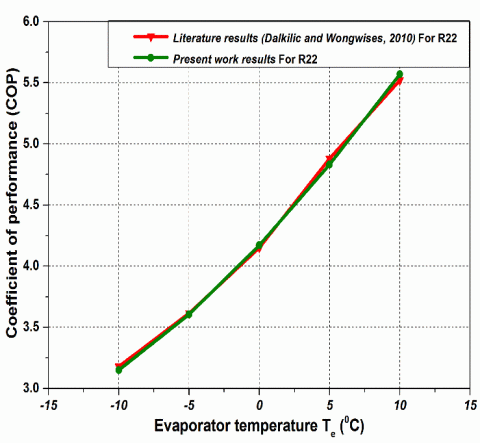Figure 5. Comparison of COP for R22 at different evaporator temperatures with literature results

## fig6.gif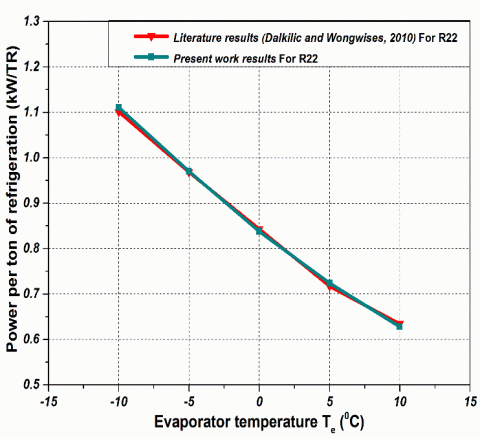Figure 6. Comparison of power per ton of refrigeration for R22 at different evaporator temperatures with literature results

## fig7.gif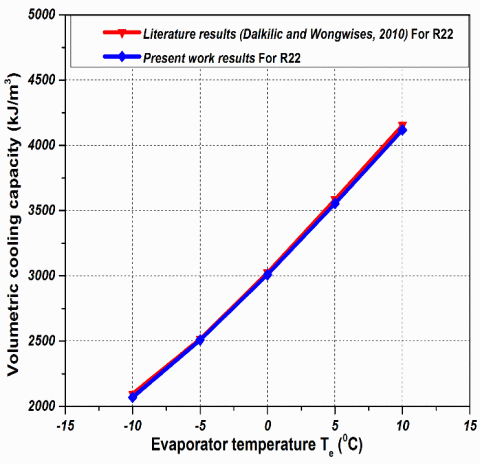Figure 7. Comparison of volumetric cooling capacity for R22 at different evaporator temperatures with literature results

6. Results and Discussions

## fig8.gif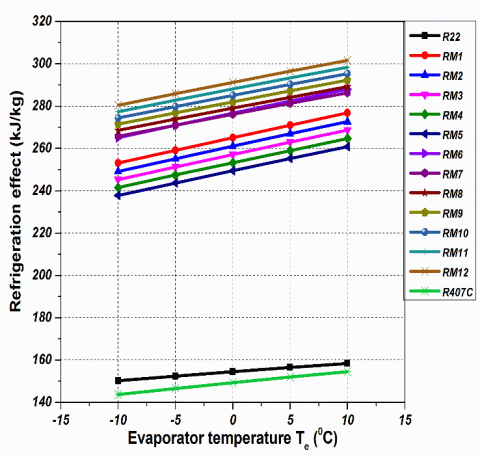Figure 8. Effect of evaporator temperature on the refrigeration effect at Tk=54.4 ℃

Figure 8 shows the effect of different evaporator temperatures on the refrigeration effect of various R22 alternatives at Tk=54.4 ℃. From Figure 8, it is noticed that the refrigeration effect increases with increase in evaporator temperature for all the investigated refrigerants. Particularly refrigeration effect of refrigerants (RM1 to RM12) is higher than R407C and R22, since conventional refrigerants are blends of hydrocarbons, which will have high latent heat of vapourization compared to R407C and R22.

6.2 Compressor work

## fig9.gif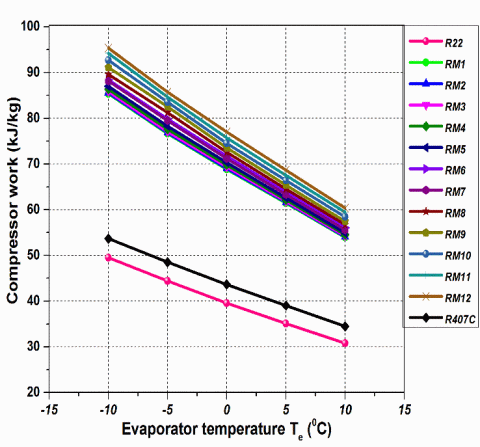Figure 9. Effect of evaporator temperature on the compressor work at Tk=54.4 ℃

Figure 9 shows the effect of different evaporator temperatures on the compressor work of various R22 alternative refrigerants at Tk=54.4 ℃. From Figure 9, it is noticed that the compressor work input decreases with increase in evaporator temperature for all the considered refrigerants. Particularly compressor work of refrigerants (RM1 to RM12) is higher than R22, since these refrigerants are blends of hydrocarbons, which will have higher vapour enthalpy compared to baseline refrigerant R22.

6.3 Coefficient of performance

## fig10.gif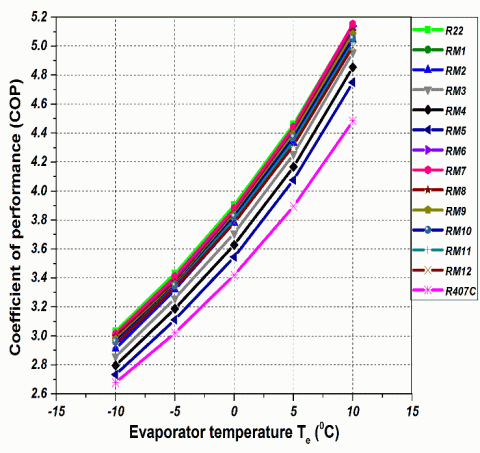Figure 10. Effect of evaporator temperature on COP at Tk=54.4 ℃

Figure 10 shows the effect of different evaporator temperatures on the coefficient of performance (COP) of various R22 alternatives at Tk=54.4 ℃. COP can be measured as an energy efficiency index of the equipment, while it is working with specific refrigerant. From Figure 10, it is evident that the COP increases with increase in evaporator temperature for all the investigated refrigerants, since COP depends upon both the refrigeration effect and work of compressor. Mainly COP of refrigerant mixture RM7 (R1270/RE170 95/5 by mass %) is the highest among twelve investigated refrigerants and it is 0.23% higher than that of COP of R22.

6.4 Pressure ratio

Figure 11 shows the effect of different evaporator temperatures on the pressure ratio of various R22 alternative refrigerants at Tk=54.4 ℃. From Figure 11, it is noticed that the pressure ratio decreases with increase in evaporator temperature for all the considered refrigerants. This is due to their increase in evaporator pressure with increase in evaporator temperature. From Figure 11 and Table 12, it is clear that the pressure ratio of twelve refrigerants studied (RM1 to RM12) is lower in the range of 0.88% to 7.94% compared to R22 whereas pressure ratio of R407C is the highest among twelve investigated refrigerants and it is 21.10% higher than R22. The high pressure ratio causes significant increase in discharge temperature of compressor.

## fig11.gifFigure 11. Effect of evaporator temperature on the pressure ratio at Tk=54.4 ℃

6.5 Compressor discharge temperature

Figure 12 shows the effect of different evaporator temperatures on the compressor discharge temperature of various R22 alternatives at Tk=54.4 ℃. From Figure 12, it is noticed that the compressor discharge temperature decreases with increase in evaporator temperature for all the investigated refrigerants, since pressure ratio of refrigerants decreases with increase in evaporator temperature. Compressor discharge temperature shows the lifetime of compressor motor and hence it is important to compute the compressor discharge temperature, while it is working with various alternative refrigerants. The excessive discharge temperature causes burnt out of compressor motor windings, which in turn reduces the lifespan of compressor significantly. Therefore compressor discharge temperature should be as low as possible from the stand point of lifespan of compressor motor is concerned. From Figure 12 and Table 12 it is evident that the compressor discharge temperature of all the twelve investigated refrigerants (RM1 to RM12) is lower in the range of 9.35 ℃ to 17.15 ℃ when compared with R22. This is due to their lower pressure ratio compared to R22 and hence refrigerants (RM1 to RM12) exhibit better durability of compressor motor.

## fig12.gif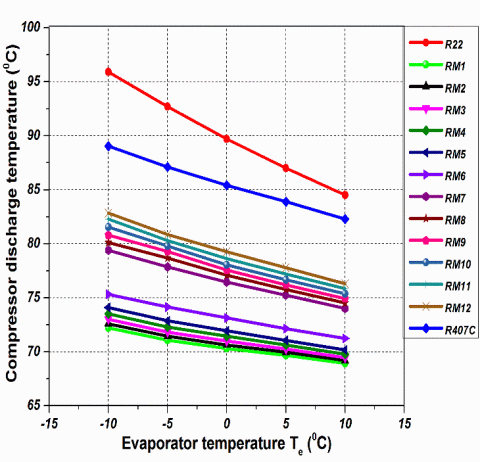Figure 12. Effect of evaporator temperature on the compressor discharge temperature at Tk=54.4 ℃

6.6 Power consumed per ton of refrigeration

## fig13.gif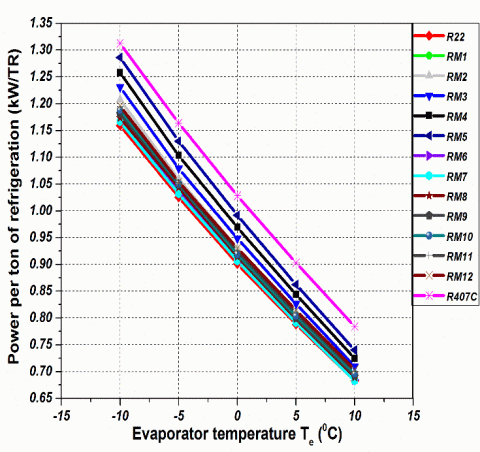Figure 13. Effect of evaporator temperature on the power per ton of refrigeration at Tk=54.4 ℃

Figure 13 shows the effect of different evaporator temperatures on the power consumed per ton of refrigeration of various R22 alternative refrigerants at Tk=54.4 ℃. It indicates the power consumed by the compressor in order to produce per ton of refrigeration and it is inversely proportional to the COP of a given refrigerant. From Figure 13, it is observed that the power consumed per ton of refrigeration decreases with increase in evaporator temperature for all the considered refrigerants, since COP of refrigerants increases with increase in evaporator temperature. From Figure 13, it is evident that the power consumed by the compressor per ton of refrigeration of refrigerant mixture RM7 (R1270/RE170 95/5 by mass %) is the lowest among twelve investigated refrigerants and it is 0.27 % lower than that of R22, since COP of RM7 is the highest among all the investigated refrigerants.

6.7 Condenser heat rejection

Figure 14 shows the effect of different evaporator temperatures on the condenser heat rejection of various R22 alternatives at Tk=54.4 ℃. From Figure 14, it is noticed that the condenser heat rejection decreases with increase in evaporator temperature for all the investigated refrigerants. Particularly condenser heat rejection of refrigerants (RM1 to RM12) is higher than R22, since these refrigerants are the blends of hydrocarbons, which will have high latent heat of condensation compared to baseline refrigerant R22.

## fig14.gif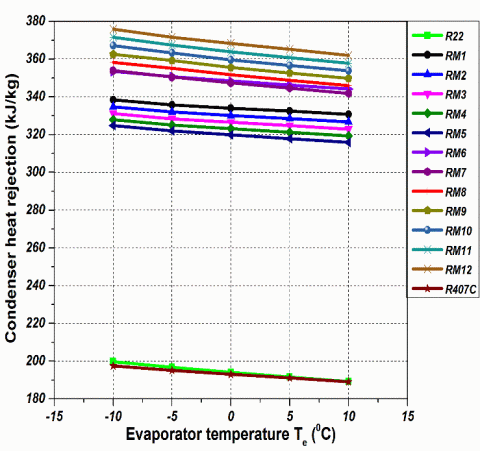Figure 14. Effect of evaporator temperature on the condenser heat rejection at Tk=54.4 ℃

6.8 Volumetric cooling capacity

Figure 15 shows the effect of different evaporator temperatures on the volumetric cooling capacity of various R22 alternative refrigerants at Tk=54.40C. It indicates the size of compressor required in order to produce desired cooling effect. Volumetric cooling capacity depends upon the vapour density occurs at the outlet of evaporator and also on the cooling effect. From Figure 15, it is observed that the volumetric cooling capacity increases with increase in evaporator temperature for all the considered R22 alternative refrigerants, since increase in volumetric capacity depends on both the values of vapour density and cooling effect of refrigerants. From Figure 15, it is evident that the volumetric cooling capacity of refrigerant mixture RM7 (R1270/RE170 95/5 by mass %) is the highest among twelve studied refrigerants and it is very close to that of volumetric capacity of R22. Therefore same size of R22 compressor can be used for RM7 without modifications.

## fig15.gifFigure 15. Effect of evaporator temperature on the volumetric cooling capacity at Tk=54.4 ℃

6.9 Heat transfer through condenser

Figure 16 shows the effect of different evaporator temperatures on the heat transfer through condenser of various R22 alternatives at Tk=54.40C. Heat transfer through condenser denotes the load taken by the condenser to reject heat to the surroundings for a given refrigerant. It depends upon both the mass flow rate and latent heat of condensation of given refrigerant. From Figure 16, it is noticed that the heat transfer through condenser decreases with increase in evaporator temperature for all the investigated refrigerants, since decrease in heat transfer depends on both the values of mass flow rate and latent heat of condensation of refrigerants. From Figure 16, it is clear that, heat transfer through condenser of refrigerant mixture RM7 (R1270/RE170 95/5 by mass %) is the lowest among twelve investigated refrigerants and it is very close to that of heat transfer through condenser of R22.

## fig16.gif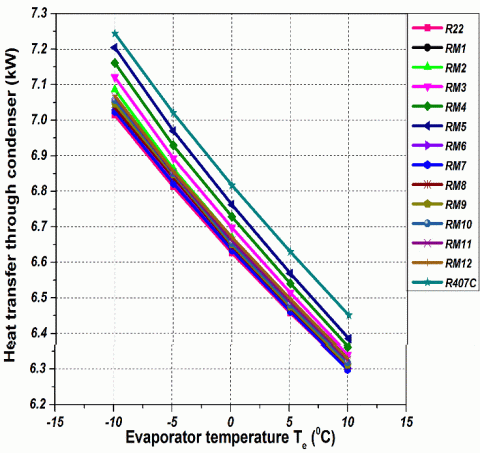Figure 16. Effect of evaporator temperature on the heat transfer through condenser at Tk=54.4 ℃

7. Conclusions

This investigation presents the theoretical thermodynamic performance analysis of window air conditioner operating with various sustainable refrigerants as substitutes to R22. The conclusions drawn from the thermodynamic analysis of various R22 alternative refrigerants are presented below.

• Coefficient of performance (COP) of refrigerant mixture RM7 (R1270/RE170 95/5 by mass %) (4.744) was the highest among twelve investigated refrigerants and it was 0.23 % higher than that of COP of R22 (4.733). Similarly COP of RM1 (R290/RE170 95/5 by mass %) (4.709) was very close to that of COP of R22 (4.733).
• Pressure ratios of all the twelve refrigerants studied (RM1 to RM12) were 0.88% to 7.49% lower when compared with R22, whereas pressure ratio of R407C was 21.10 % higher than that of R22. Specifically pressure ratio of RM7 was 7.49% lower than that of R22.
• Compressor discharge temperatures of all the twelve investigated refrigerants (RM1 to RM12) were 9.35 ℃ to 17.15 ℃ lower than that of discharge temperature of R22. Therefore all the twelve investigated refrigerants exhibit better lifetime and durability of compressor motor. Specifically discharge temperature of RM1 was 17.15 ℃ lower compared to R22.
• Power consumed per ton of refrigeration of RM7 (0.741 kW/TR) was the lowest among twelve investigated refrigerants and it was 0.27 % lower than that of power per ton of refrigeration of R22 (0.743 kW/TR). Similarly power consumed per ton of refrigeration of RM1 (0.746 kW/TR) was slightly higher than that of R22 (0.743 kW/TR).
• Volumetric cooling capacity of RM7 (3833 kJ/m3) was the highest among twelve refrigerants studied and it was closer to that of R22 volumetric capacity (3868 kJ/m3). Therefore same size of R22 compressor would be used for RM7 without modifications.
• Heat transfer through condenser of RM7 (6.372 kW) was the lowest among twelve refrigerants studied and it was closer to that of R22 (6.377 kW).
• Flammability investigation revealed that all the twelve investigated refrigerants were categorized into ASHRAE A3, flammable group. Therefore, safety precautions must be followed while using these refrigerants.
• Global warming potential (GWP100) value of all the twelve refrigerants studied (RM1 to RM12) (<3) were very low when compared to that of GWP100 of R22 (1760).
• From the thermodynamic performance investigation of various studied sustainable R22 alternatives, it was concluded that the refrigerant mixture RM7 (R1270/RE170 95/5 by mass %) could be an appropriate sustainable alternative to R22 used in air conditioners, from the view point of COP, discharge temperature, GWP, ODP, power consumption, pressure ratio, and volumetric capacity.
Nomenclature
 BP Boiling point, ℃ COP Coefficient of performance, Dimensionless CHR Condenser heat rejection, kJ/kg HOC Enthalpy of combustion, kJ/mol L Lower flammability limit, kg/m3 MW Molecular weight, kg/kmol PPTR Power required per ton of refrigeration, kW/TR RE Refrigeration effect, kJ/kg TR Ton of refrigeration, kW U Upper flammability limit, kg/m3 VCC Volumetric cooling capacity, kJ/m3 Cp0 Ideal gas heat capacity, J/mol K h Enthalpy, kJ/kg hf Liquid enthalpy, kJ/kg hfg Enthalpy of vapourization, kJ/kg hg Vapour enthalpy, kJ/kg h1” Enthalpy at compressor inlet, kJ/kg h2” Enthalpy at compressor outlet, kJ/kg h3” Enthalpy at condenser outlet, kJ/kg h4” Enthalpy at evaporator inlet, kJ/kg P Pressure, MPa Pc Critical pressure, Mpa Pcm Critical pressure of mixture, MPa Pe Evaporating pressure, MPa Pk Condensing pressure, MPa Pr Pressure ratio, Dimensionless Psat Saturation pressure, MPa Qc Refrigeration capacity, kW Qk Heat transfer through condenser, kW R Universal gas constant, J/mol K Sf Liquid entropy, kJ/kg K Sfg Entropy of vapourization, kJ/kg K Sg Vapour entropy, kJ/kg K T Temperature, K Tbub Bubble point temperature, 0C Tc Critical temperature, K Tcm Critical temperature of mixture, K Tdew Dew point temperature, ℃ Te Evaporating temperature, ℃ Tglide Temperature glide, ℃ Tk Condensing temperature, ℃ Tsat Saturation temperature, K U Internal energy, kJ/kg V Specific volume, m3/kg Vcm Critical volume of mixture, m3/kg Vg Vapour volume, m3/kg Vf Liquid volume, m3/kg Wc Specific work of compressor, kJ/kg Zcm Critical compressability factor of mixture, Dimensionless ρ Density, kg/m3 ρc Critical density, kg/m3 ω Accentric factor, Dimensionless Greek symbols ρ Density, kg/m3 ω Accentric factor, Dimensionless Subscripts c Critical f Liquid phase g Vapour phase m Mixture Abbreviations AHRI Air-Conditioning, Heating, and Refrigeration    Institute ASHRAE American Society of Heating, Refrigerating and Air-Conditioning Engineers BP Boiling point CHR Condenser heat rejection COP Coefficient of performance GWP Global warming potential HCFCs Hydrochlorofluorocarbons HFO Hydrofluoroolefin MHEOS Martin-Hou equation of state ODP Ozone depleting potential PPTR Power required per ton of refrigeration RE Refrigeration effect RF Refrigerant flammability TR Ton of refrigeration VCC Volumetric cooling capacity VCR Vapour compression refrigeration
References

 Mohanraj M, Jayaraj S, Muraleedharan C. (2009). Environment friendly alternatives to halogenated Refrigerants-A review. International Journal of Greenhouse /Gas Control 3(1): 108-119. https://doi.org/10.1016/j.ijggc.2008.07.003

 United Nations Environmental Programme (1987). Montreal Protocol on substances that deplete the ozone layer Final act, New York, United Nations.

 Powell RL. (2002). CFC Phase out; have we met the challenge. Journal of Fluorine Chemistry 114(2): 237-250. https://doi.org/10.1016/S0022-1139(02)00030-1

 Afshari F, Comakli O, Adiguzel N, Karagoz S. (2016). Optimal charge amount for different refrigerants in air-to-water heat pumps. Iranian Journal of Science and Technology, Transaction of Mechanical Engineering 40(4): 325-335. ttps://doi.org/10.1007/s40997-016-0028-2

 Bolaji BO, Adeleke AE, Adu MR, Olanipekun MU, Akinnibosun E. (2019). Theoretical investigation of energy-saving potential of eco-friendly R430A, R440A and R450A refrigerants in a domestic refrigerator. Iranian Journal of Science and Technology, Transaction of Mechanical Engineering 43(1): 103-112. https://doi.org/10.1007/s40997-017-0110-4

 Elraheim DA, Mahmoud OE, Fatouh M. (2016). Experimental assessment of a domestic split type air-conditioner working with R410A. International Journal of Air-Conditioning and Refrigeration 24(4): 1650021. https://doi.org/10.1142/S2010132516500218

 Mota-Babiloni A, Navarro-Esbrí J, Makhnatch P, Molés F. (2017). Refrigerant R32 as lower GWP working fluid in residential air conditioning systems in Europe and the USA. Renewable and Sustainable Energy Review 80: 1031-1042. https://doi.org/10.1016/j.rser.2017.05.216

 Shen B, Bhandari M, Rane M, Mota D. (2016). Low GWP refrigerants modelling study for a room air conditioner having microchannel heat exchangers. International Refrigeration and Air Conditioning Conference, Purdue, pp. 1-9.

 Bolaji BO, Abiala IO, Ismaila SO, Borokinni FO. (2014). Theoretical comparison of two eco-friendly refrigerants as alternatives to R22 using a vapour compression refrigeration system. Transactions of Famena 38(3): 59-70. https://hrcak.srce.hr/129615

 Devotta S, Waghmare AV, Sawant NN, Domkundwar BM. (2001). Alternatives to HCFC-22 for air conditioners. Applied Thermal Engineering 21(6): 703-715. https://doi.org/10.1016/S1359-4311(00)00079-X

 Arora A, Kaushik SC. (2008). Theoretical analysis of a vapour compression refrigeration system with R502, R404A and R507A. International Journal of Refrigeration 31(6): 998-1005. https://doi.org/10.1016/j.ijrefrig.2007.12.015

 Bolaji BO, Huan Z. (2012). Energy performance of eco-friendly RE170 and R510A refrigerants as alternatives to R134a in vapour compression refrigeration system. Proceedings of the 9th Industrial and Commercial Use of Energy Conference, Stellenbosch, South Africa, pp. 139-146.

 Antunes AHP, Bandarra Filho EP. (2016). Experimental investigation on the performance and global environmental impact of a refrigeration system retrofitted with alternative refrigerants. International Journal of Refrigeration 70: 119-127. https://doi.org/10.1016/j.ijrefrig.2016.06.027

 Wu Y, Liang X, Tu X, Zhuang R. (2012). Study of R161 refrigerant for residential air-conditioning applications. International Refrigeration and Air Conditioning Conference, Purdue, USA, pp. 1-7.

 Kim NH. (2016). Application of the natural refrigerant mixture R-290/DME to a soft ice cream refrigerator. International Journal of Air-Conditioning and Refrigeration 24(4): 1650027. https://doi.org/10.1142/S2010132516500279

 Park KJ, Shim YB, Jung D. (2009). Experimental performance of R432A to replace R22 in residential air-conditioners and heat pumps. Applied Thermal Engineering 29(2-3): 597-600. https://doi.org/10.1016/j.applthermaleng.2008.02.019

 Datta SP, Das PK, Mukhopadhyay S. (2016). Performance of a condenser of an automotive air conditioner with maldistribution of inlet air-Simulation studies and its experimental validation. International Journal of Heat and Mass Transfer 98: 367-379. https://doi.org/10.1016/j.ijheatmasstransfer.2016.03.019

 Cho H, Park C. (2016). Experimental investigation of performance and exergy analysis of automotive air conditioning systems using refrigerant R1234yf at various compressor speeds. Applied Thermal Engineering 101: 30-37. https://doi.org/10.1016/j.applthermaleng.2016.01.153

 Meng Z, Zhang H, Lei M, Qin Y, Qiu J. (2018). Performance of low GWP R1234yf/R134a mixture as a replacement for R134a in automotive air conditioning systems. International Journal of Heat and Mass Transfer 116: 362-370. https://doi.org/10.1016/j.ijheatmasstransfer.2017.09.049

 Wongwises S, Kamboon A, Orachon B. (2006). Experimental investigation of hydrocarbon mixtures to replace HFC-134a in an automotive air conditioning system. Energy Conversion and Management 47(11-12): 1644-1659. https://doi.org/10.1016/j.enconman.2005.04.013

 Harby K. (2017). Hydrocarbons and their mixtures as alternatives to environmental unfriendly halogenated refrigerants: An updated overview. Renewable and Sustainable Energy Reviews 73: 1247-1264. https://doi.org/10.1016/j.rser.2017.02.039

 Sarbu I. (2014). A review on substitution strategy of non-ecological refrigerants from vapour compression-based refrigeration, air-conditioning and heat pump systems. International Journal of Refrigeration 46: 123-141. https://doi.org/10.1016/j.ijrefrig.2014.04.023

 ASHRAE. (2017). ASHRAE handbook– fundamentals American Society of Heating, Refrigerating and Air-Conditioning Engineers, Atlanta, USA.

 Martin JJ, Hou YC. (1955). Development of an equation of state for gases. AIChE Journal 1(2): 142-151. https://doi.org/10.1002/aic.690010203

 De Monte F. (2002a). Calculation of thermodynamic properties of R407C and R410A by the Martin–Hou equation of state - part I: theoretical development. International Journal of Refrigeration 25(3): 306-313. https://doi.org/10.1016/S0140-7007(01)00028-7

 De Monte F. (2002b). Calculation of thermodynamic properties of R407C and R410A by the Martin–Hou equation of state - part II: technical interpretation. International Journal of Refrigeration 25(3): 314-329. https://doi.org/10.1016/S0140-7007(01)00029-9

 Brown JS, Fedele L, Nicola GDi. (2015). Compressed liquid density and vapor phase PvT measurements of cis-1,2,3,3,3-pentafluoroprop-1-ene (R1225ye(Z)). Journal of Chemical Engineering & Data 60(11): 3333-3340. https://doi.org/10.1021/acs.jced.5b00562

 Nicola GDi, Brown JS, Fedele L, Securo M, Bobbo S, Zillo C. (2013). Subcooled liquid density measurements and PvT measurements in the vapor phase for 3,3,3-trifluoroprop-1-ene (R1243zf). International Journal of Refrigeration 36(8): 2209-215. https://doi.org/10.1016/j.ijrefrig.2013.08.004

 Brown JS, Nicola GD, Zillo C, Fedele L, Bobbo S, Polonara F. (2012). Subcooled liquid density measurements and PvT measurements in the vapor phase for trans-1,3,3,3-Tetrafluoroprop-1-ene (R1234ze(E)). Journal of Chemical & Engineering Data 57(12): 3710-3720. https://doi.org/10.1021/je300945r

 Nicolla CDi, Nicolla GDi, Paceti M, Polonara F, Santori G. (2010). P-V-T behavior of 2,3,3,3-tetrafluoroprop-1-ene (HFO-1234yf) in the vapor phase from (243 to 373) K. Journal of Chemical and Engineering Data 55(9): 3302-3306. https://doi.org/10.1021/je100102q

 Dong L, Zhang Y, Li S, Wei S, Zhang J, Qi Y. (2012). An empirical equation to directly calculate parameter B4 of the Martin-Hou equation of state. Chemical Engineering Communication 199(5): 577-586. https://doi.org/10.1080/00986445.2011.599899

 Arora CP. (2009). Refrigeration and air conditioning. Tata McGraw-Hill, New Delhi.

 Poling BE, Prausnitz JM, O’Connell JP. (2001). The Properties of Gases and Liquids. McGraw-Hill, New York.

 Forero GLA, Velásquez JJA. (2011). Wagner liquid-vapour pressure equation constants from a simple methodology. The Journal of Chemical Thermodynamics 43(8):  1235-1251. https://doi.org/10.1016/j.jct.2011.03.011

 Nasrifar K, Moshfeghian M. (1999). Evaluation of saturated liquid density prediction methods for pure refrigerants. Fluid Phase Equilibria 158: 437-445. https://doi.org/10.1016/S0378-3812(99)00068-0

 Lemmon EW, Huber ML, McLinden MO. (2013). NIST Reference Fluid Thermodynamic and Transport Properties-REFPROP, NIST Standard Reference Database 23-Version 9.1.

 ANSI/ASHRAE for Designation and Safety Classification of Refrigerants (2007). ANSI/ASHRAE Standard 34.

 Kondo S, Takahashi A, Tokuhashi K, Sekiya A. (2002). RF number as a new index for assessing combustion hazard of flammable gases. Journal of Hazardous Materials 93(3): 259-267. https://doi.org/10.1016/S0304-3894(02)00117-6

 Bolaji BO, Huan Z. (2012). Comparative analysis of the performance of hydrocarbon refrigerants with R22 in a sub-cooling heat exchanger refrigeration system. Proceedings of the Institution of Mechanical Engineers, Part A: Journal of Power and Energy 226(7): 882-891. https://doi.org/10.1177/0957650912454829

 Dalkilic AS, Wongwises S. (2010). A performance comparison of vapour-compression refrigeration system using various alternative refrigerants. International Communications in Heat Mass Transfer 37(9): 1340–1349. https://doi.org/10.1016/j.icheatmasstransfer.2010.07.006

 Bolaji BO, Huan Z. (2012). Computational analysis of the performance of ozone-friendly R22 alternative refrigerants in vapour compression air-conditioning. Environment ‎Protection Engineering 38(4): 41-52. https://doi.org/10.5277/EPE120404

 Bolaji BO, Huan Z. (2014). Performance investigation of some hydro-fluorocarbon refrigerants with low global warming as substitutes to R134a in refrigeration systems. Journal of Engineering Thermophysics 23(2): 148–157. https://doi.org/10.1134/S1810232814020076